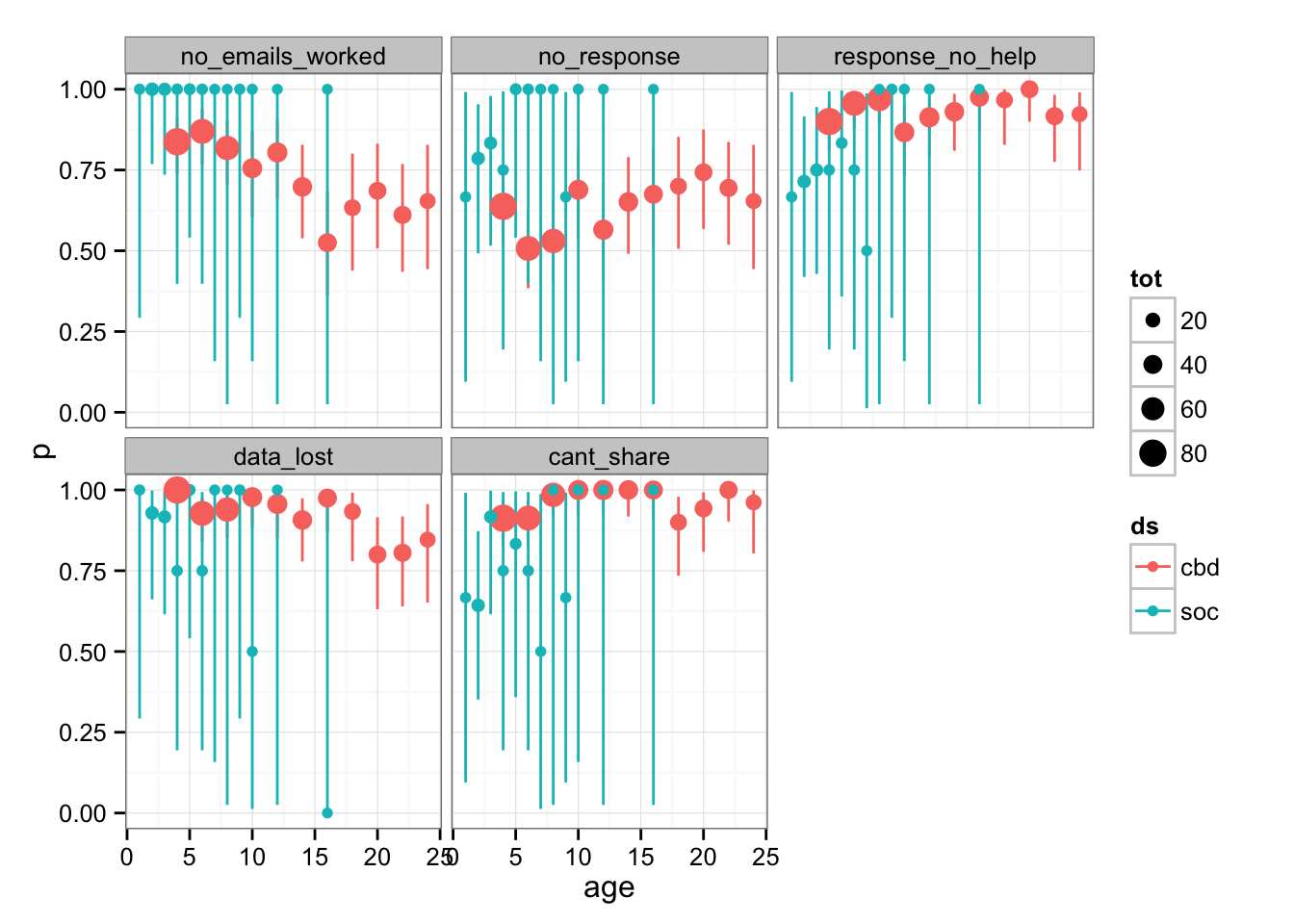Combining information from Vines et al. (2014) and Young (2015) to look at various causes of non-reproducibility. (This is just a preliminary hack at gathering and munging the data.)

library("gdata")  ## for read.xls()
library("dplyr")
library("tidyr")
library("ggplot2"); theme_set(theme_bw())
url1 <- "http://datadryad.org/bitstream/handle/10255/dryad.56139/CurrentBiologyData.txt?sequence=1"
if (!file.exists("CurrentBiologyData.txt")) {
}
url2 <- "http://www.stanford.edu/~cy10/public/data/ReplicationData_deidentified.xls"
if (!file.exists("sociologists.xls")) {
}
socd <- read.xls("sociologists.xls")

Modify the sociology data to match Vines et al. format:

no_help_responses <- c("data still in use by author",
"too complicated", "timeframe issue",
"'see the article and figure it out'")

socd2 <- socd %>% select(-JOURNAL) %>%
rename(rs=RESPONSE_SPECIFIC,rg=RESPONSE_GENERAL) %>%
transmute(year=YEAR_PUBLISHED,
ds="soc",
no_emails_worked=0,
no_response=as.numeric(rg=="No Response"),
response_no_help=as.numeric(rs %in% no_help_responses),
data_lost=as.numeric(rs=="don't have data"),
cant_share=as.numeric(rs=="IRB/legal/confidentiality issue"))

Clean up/select the Current Biology data:

cbd2 <- cbd %>% select(year,no_emails_worked,no_response,
response_no_help,data_lost,cant_share) %>%
mutate(ds="cbd")

Utility function for binomial confidence intervals:

get_binCI  <- function(x,n) {
bi <- binom.test(x,n)$conf.int data_frame(lwr = bi,upr = bi) } comb <- rbind(cbd2,socd2) Lcomb <- comb %>% gather(var,result,-year,-ds) Lcomb2 <- Lcomb %>% group_by(year,ds,var) %>% summarise( tot=length(result), n=sum(1-result), p=mean(1-result)) %>% group_by(year,ds,var,n,tot) %>% do(cbind(.,get_binCI(.$n,.\$tot))) %>%
ungroup() %>%
mutate(age=2015-year)

Notes

• this plot does not replicate the Current Biology plot, which rather than computing the probability per age of each outcome instead computes the conditional probabilities (e.g. prob(useful response|any response at all)) …
• the facet labels are misleading: these are the probabilities of response, shareability, etc. (see the 1-result transformation in the pipeline above), in order to match the Current Biology plots which showed “chance of success”. The facet labels represent the failure modes (e.g. cant_share) but I haven’t changed the factor levels accordingly …
• the sociology data set had smaller samples (smaller points/larger CIs) and seemed to be conditioned on working e-mail addresses (i.e. they didn’t give a category equivalent to no_emails_worked, but these may have been lumped into the “no response” category)
ggplot(Lcomb2,
aes(age,p,colour=ds)) +
geom_linerange(aes(ymin=lwr,ymax=upr)) +
geom_point(aes(size=tot))+
scale_size(range=c(2,5))+
facet_wrap(~var)## References

Vines, Timothy H., Arianne Y.K. Albert, Rose L. Andrew, Florence Débarre, Dan G. Bock, Michelle T. Franklin, Kimberly J. Gilbert, Jean-Sébastien Moore, Sébastien Renaut, and Diana J. Rennison. 2014. “The Availability of Research Data Declines Rapidly with Article Age.” Current Biology 24 (1): 94–97. doi:10.1016/j.cub.2013.11.014.

Young, Cristobal. 2015. “Sociologists Need to Be Better at Replication.” Orgtheory.net. https://orgtheory.wordpress.com/2015/08/11/sociologists-need-to-be-better-at-replication-a-guest-post-by-cristobal-young/.